Homogeneous space of an algebraic group

An algebraic varietytogether with a regular transitive action of an algebraic groupgiven on it. If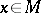, then the isotropy group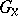is closed in. Conversely, ifis a closed subgroup of an algebraic group, then the set of left cosets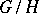has the structure of an algebraic variety, making it into a homogeneous space of the algebraic group, where the natural mapping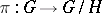is regular, separable and has the following universal property: For any morphism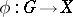constant on cosets, there is a morphism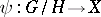such that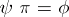. Ifis any homogeneous space of the algebraic groupandfor some, then the natural bijection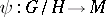is regular, and if the ground fieldhas characteristic 0, thenis biregular (see , ).
Suppose that the connected group, the homogeneous spaceand the action ofonare defined over some subfield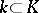. Then the group of-rational points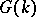takes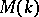into itself and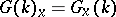for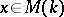. Ifis finite, then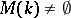, and if moreover the isotropy groupis connected, thenacts transitively on. In the general case, the study of the-rational points inreduces to problems in the theory of Galois cohomology (see ).
A homogeneous space of an algebraic groupis always a smooth quasi-projective variety (see ). Ifis an affine algebraic group, then the varietyis projective if and only ifis a parabolic subgroup in(see ). Ifis reductive, then the varietyis affine if and only if the subgroupis reductive (see Matsushima criterion). A description is also known of the closed subgroupsof a linear algebraic groupover an algebraically closed field of characteristic 0 for whichis quasi-affine (see , ).